# 31.3: Transition-Metal Compounds as Reagents for Organic Syntheses

## Reactions of Zirconocene Chlorohydride

Some transition-metal hydrides show promise as synthetic reagents of the same general applicability as the boron hydrides (Section 11-6). An excellent illustration is provided by the work of J. Schwarz with zirconocene chlorohydride, $$13$$, which is available by reduction of zirconocene dichloride: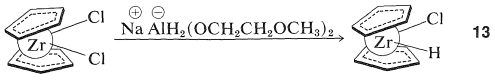(The cyclopentadienide rings in $$13$$ are shown as being nonparallel and this is in accord with x-ray diffraction studies of metallocenes that have extra substituents on the metal.) Henceforth we will abbreviate the structure $$13$$ by $$\ce{(Cp)_2ZrClH}$$.

Alkenes react with $$\ce{(Cp)_2ZrClH}$$ to form alkyl-$$\ce{Zr}$$ bonds with zirconium becoming attached to the least-hindered primary carbon: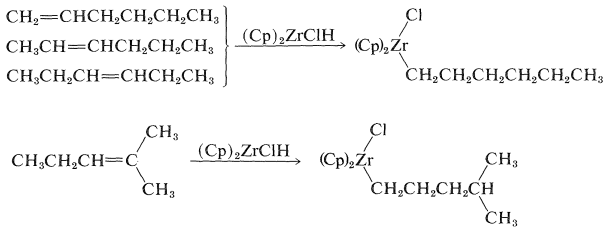The initial step in this kind of reaction is formation of the $$\pi$$-alkene complex followed by hydride transfer: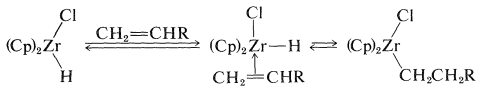These reaction must be reversible for an alkene with an internal double bond to form an adduct with the metal atom at the end of the chain. The process is seen as a series of interconversions between $$\pi$$ and $$\sigma$$ complexes, which permits the metal atom to move to the least-hindered (primary) carbon:Figure 31-1. Similar reactions also can be carried out with alkynes by way of complexes such as $$\ce{(Cp)_2Zr(Cl)CH=CH_2}$$.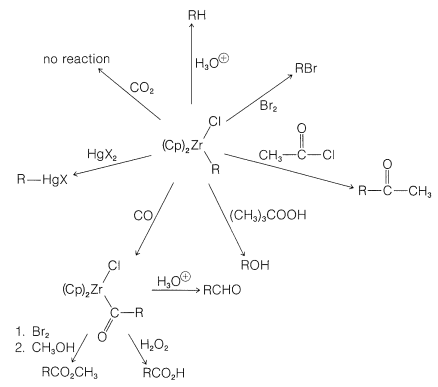Figure 31-1: Synthetic reactions of alkylzirconocenes. In general, the reactions parallel those of the boranes (Sections 11-6 and 16-9G).

One of the elegant features of these reactions is the formation of crystalline $$\ce{[(Cp)_2Zr(Cl)]_2O}$$ on treatment of the reaction products with water. This substance can be converted back to zirconocene dichloride with $$\ce{HCl}$$ and thence back to $$\ce{(Cp)_2ZrClH}$$: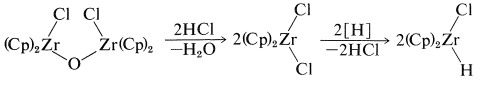Figure 31-1. This reaction proceeds by way of a carbon-monoxide complex of the metal, which then rearranges by an alkyl shift:This sequence of steps is an important part of the mechanism of the hydroformylation of alkenes (oxo reaction), to be discussed in Section 31-4B, and also is related to the carbonylation reactions of boranes discussed in Section 16-9G.

## A Nucleophilic Transition-Metal Reagent. Sodium Tetracarbonylferrate(-II)

Sodium reacts with iron pentacarbonyl to produce a salt known as sodium tetracarbonylferrate(-II)$$^2$$, $$\ce{Na_2Fe(CO)_4}$$, which has been shown by J. P. Collman and co-workers to have considerable potential as a reagent for organic synthesis.

$2 \ce{Na} + \ce{Fe(CO)_5} \rightarrow \ce{Na_2Fe(CO)_4} + \ce{CO}$

The tetracarbonylferrate dianion is a good nucleophile and reacts with alkyl halides or alkyl sulfonate esters by the $$S_\text{N}2$$ mechanism (with inversion) to form $$\ce{C-Fe}$$ bonds:The resulting anion undergoes insertion with carbon monoxide or ketone formation with acyl halides in a manner similar to alkylchlorozirconocenes (Section 31-3A):The product of $$\ce{CO}$$ insertion has the potential of transferring $$\ce{R}- \overset{\ominus}{\ce{C}} \ce{=O}$$, and is converted to $$\ce{RCHO}$$ with acids, to $$\ce{RCOX}$$ with halogens, or to $$\ce{RCO_2H}$$ by oxidation:$$^2$$The designation (-II) indicates that the iron in this substance can be regarded as being in the -2 oxidation state.